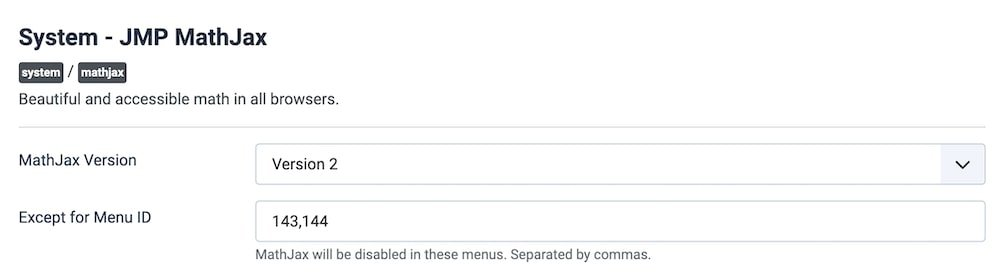# Joomla plugin for displaying mathematical formulas

This plugin inherits the MathJax library, a tool used to display math formulas on all browsers. MathJax is highly modular on input and output. Use MathML, TeX, and ASCIImath as input and produce HTML+CSS, SVG, or MathML as output.

It works with Joomla 2.5+, 3 and 4.

After installing the plugin, GOTO the Plugins > System - JMP MathJax > Enable it.

In order to disable MathJax for some specific pages, add the Menu IDs to Except for Menu ID (Separated by commas).Demo:

When $$a \ne 0$$, there are two solutions to $$ax^2 + bx + c = 0$$ and they are
$$x = {-b \pm \sqrt{b^2-4ac} \over 2a}.$$

---------------

### You may also like

#### File Manager Extension for Joomla